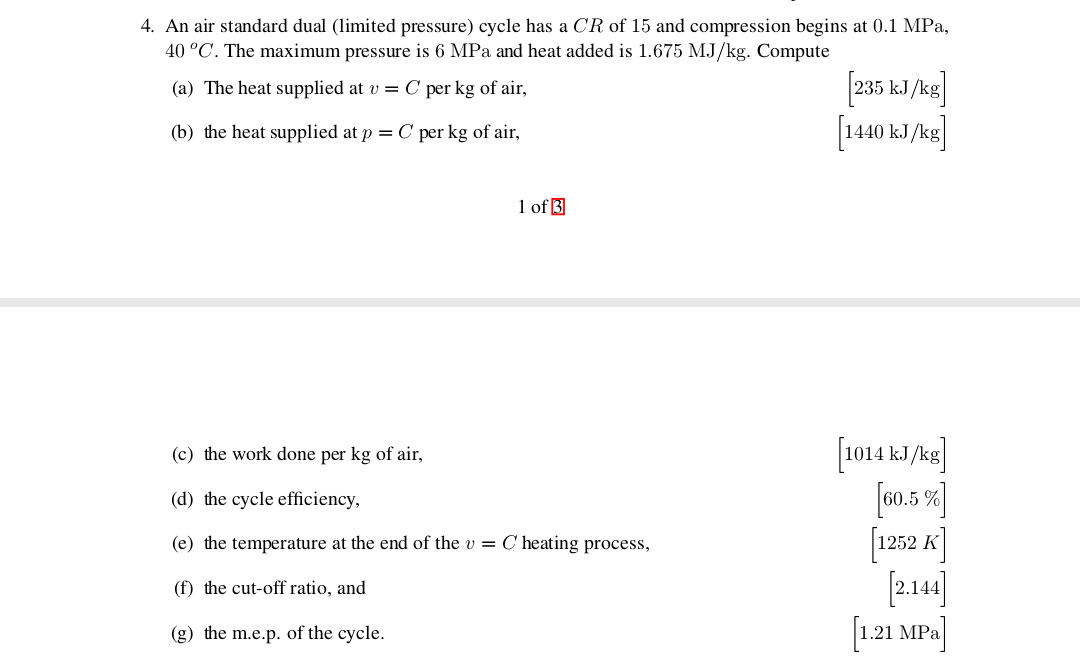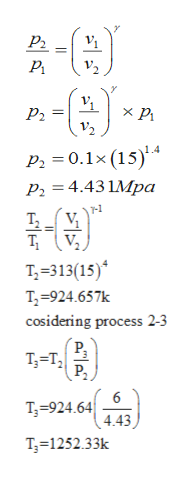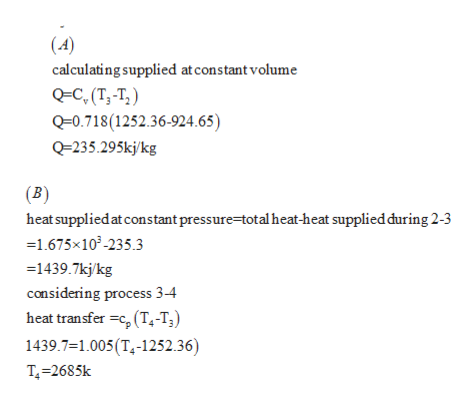# 4. An air standard dual (limited pressure) cycle has a CR of 15 and compression begins at 0.1 MPa,40 °C. The maximum pressure is 6 MPa and heat added is 1.675 MJ/kg. Compute235 kJkg1440 kJ/k(a) The heat supplied at v = C per kg of air(b) the heat supplied at p = C per kg of air1 of 31014 k/kg]0.5%(c) the work done per kg of air,(d) the cycle efficiency(e) the temperature at the end of the v = C heating process,(f) the cut-off ratio, andL21 MP(g) the m.e.p. of the cycle.

Question
21 viewshelp_outlineImage Transcriptionclose4. An air standard dual (limited pressure) cycle has a CR of 15 and compression begins at 0.1 MPa, 40 °C. The maximum pressure is 6 MPa and heat added is 1.675 MJ/kg. Compute 235 kJkg 1440 kJ/k (a) The heat supplied at v = C per kg of air (b) the heat supplied at p = C per kg of air 1 of 3 1014 k/kg] 0.5% (c) the work done per kg of air, (d) the cycle efficiency (e) the temperature at the end of the v = C heating process, (f) the cut-off ratio, and L21 MP (g) the m.e.p. of the cycle. fullscreen
check_circle

Step 1

Hii there thanks for the question as per our honor code we are authorized to solve only three subparts of question. Please resubmit your question for the other subparts.

Given data as per question

Step 2

Considering process 1-2help_outlineImage TranscriptioncloseР. J V2 P 0.1x (154 P2 4.43 1Mpa T-313(15) T-924.657k cosidering process 2-3 T T Р, 6 T924.64 4.43 T3 1252.33k fullscreen
Step 3help_outlineImage Transcriptionclose(4) calculating supplied at constant volume QC, (T,-T;) Q-0.718(1252.36-924.65) Q=235.295kjkg (B) heat suppliedat constant pressure=total heat-heat supplied during 2-3 =1.675x103-235.3 - 1439.7kj/kg considering process 3-4 heat transfer c (T4-T3) 1439.7=1.005(T4-1252.36) T-2685k fullscreen

### Want to see the full answer?

See Solution

#### Want to see this answer and more?

Solutions are written by subject experts who are available 24/7. Questions are typically answered within 1 hour.*

See Solution
*Response times may vary by subject and question.
Tagged in

### Mechanical Engineering